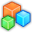# Microsoft Small BasicProgram Listing: VWH504
' SmallBasic Version 1.2
' Program: RemaindersChall
' Changelog:
' Author: Pappa Lapub
' Website: https://social.msdn.microsoft.com/Forums/en-US/65e3624b-5700-4bc8-9b62-e67c6bb4e62d/challenge-of-the-month-november-2018
' ImportURL: http://smallbasic.com/program/?
' SBOnline: http://smallbasic-publicwebsite-dev.azurewebsites.net/Program/view.aspx?
' Extension: ---
' Comment:
'
' Variables:
' ToDo:
' ==============================================================================
' 1. What is the smallest number which when divided by 28 leaves 8 as remainder and when divided by 32 leaves 12 as the remainder?
While res = ""
x = x + 4 ' 1 ; 4 = gcd(8,12)
If (Math.Remainder(x,28) = 8) And (Math.Remainder(x,32) = 12) Then
res = x
EndIf
EndWhile
TextWindow.WriteLine("x1 = "+ x) ' 204

' 2. What is the smallest four digit number which when divided by 16 and 18 leaves remainder 8 in each case?
x = 992 ' 999
res = ""
While res = "" And x < 10000
x = x + 8 ' 1
If (Math.Remainder(x,16) = 8) And (Math.Remainder(x,18) = 8) Then
res = x
EndIf
EndWhile
TextWindow.WriteLine("x2 = "+ x) ' 1016

' 3. What is the smallest number which when divided by 12 and 18 leaves a remainder of 9?
x = 0
res = ""
While res = ""
x = x + 9 ' 1
If (Math.Remainder(x,12) = 9) And (Math.Remainder(x,18) = 9) Then
res = x
EndIf
EndWhile
TextWindow.WriteLine("x3 = "+ x) ' 9

' 4. What is the smallest number that leaves 3 as remainder when divided by 18 and 24?
x = 0
res = ""
While res = ""
x = x + 3 ' 1
If (Math.Remainder(x,18) = 3) And (Math.Remainder(x,24) = 3) Then
res = x
EndIf
EndWhile
TextWindow.WriteLine("x4 = "+ x) ' 3

' 5. What is the smallest number which when divided by 14 and 21 leaves no remainder?
x = 0
res = ""
While res = ""
x = x + 1
If (Math.Remainder(x,14) = 0) And (Math.Remainder(x,21) = 0) Then
res = x
EndIf
EndWhile
TextWindow.WriteLine("x5 = "+ x) ' 42 = 3*14 = 2*21 = lcm(14,21)

' 6. What is the smallest number which leaves 3 as a remainder when divided by 9, 12, 18, and 30?
x = 0
res = ""
While res = ""
x = x + 3 ' 1
If (Math.Remainder(x,9) = 3) And (Math.Remainder(x,12) = 3) And (Math.Remainder(x,18) = 3) And (Math.Remainder(x,30) = 3) Then
res = x
EndIf
EndWhile
TextWindow.WriteLine("x6 = "+ x) ' 3

'x1 = 204
'x2 = 1016
'x3 = 9
'x4 = 3
'x5 = 42
'x6 = 3